# Mathematics - Signals And Systems - Laplace Transform[Image1]

## Introduction

Hey it's a me again @drifter1!

Today we continue with my mathematics series about Signals and Systems in order to cover the Laplace Transform.

So, without further ado, let's dive straight into it!

## Getting into the Laplace Transform

Up to this point in the series linear time-invariant (LTI) system analysis has been based on the properties of Fourier analysis. The properties of this Fouriers analysis are a direct consequence of representing signals as linear combinations of basic signals. Choosing the eigenfunctions of LTI systems to be of the form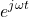led to the Fourier transform synthesis equation. And using this representation any given LTI system can be represented by the spectrum of eigenvalues as a function of ω, which is basically the ampltiude change that the system applied to each of those basic inputs.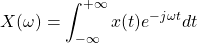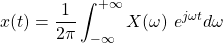But, the Fourier transform comes with restrictions. Thus, in order to generalize the Fourier transform for a broader class of signals and systems that it doesn't converge to, the Laplace transform was born. The Laplace transform is using eigenfunctions which are complex exponentials of the form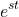, where s is a complex number. Using this more general class allows for the representation of signals and systems in terms of the Laplace transform. The response of an LTI system to a complex exponential of the formis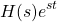.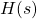represents the change in amplitude, and is referred to as the system function. In other words,is the Laplace transform of the system impulse response.

Of course the Laplace and Fourier transform are closely related to each other. The variable s can be thought of as. Therefore, when s is purely imaginary or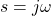, the Laplace transform is basically reduced to the Fourier transform. So, the Laplace transform is the Fourier transform of a signal after an exponential weighting ofhas been applied. Because of that, the Laplace transform converges for signals for which the Fourier transform does not converge.

## Laplace Transform

The Laplace transform is used for continuous-time signals and systems. Mathematically, the following changes can be applied to Fourier transform equation: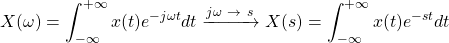Similar to the Fourier transform the Laplace transform is also represented mathematically using the first letter in its name, which is L, and as such: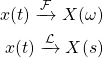Using the Laplace transform, the impulse response (system response for complex exponential) is:Using the definition of s as, we can re-write the equation of the Laplace transform as follows:As such, the Laplace transform is basically the Fourier transform of, or mathematically:
This also explains why one might converge, whilst the other may not.

### Example

Let's take the commonly used exponential:as an quick example.

From the Fourier transform pairs, we know that its Fourier transform is: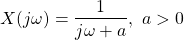We changed the notation in order to make it easier to relate the Fourier transform with the Laplace transform, meaning that now:Well, because, when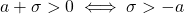, the Laplace transform is similar and simply:With this example we quietly introduced the concept of convergence.

## Region of Convergence (ROC)

From what we saw up to this point, the Laplace transform is a function of a general complex variable s, which converges for a range of values. This range is referred to as the region of convergence (ROC) and is very important in associating the Laplace transform with a given signal. When talking about the Fourier transform any given signal had a unique algebraic expression, but in the case of the Laplace transform two different signals might have an identical algebraic expression, but differ at the ROC. So, in the case of the Laplace transform a signal is represented by both its mathematical expression and the range of values for which s is valid.

An easy way to deal with signals when using the Laplace transform is by representing them as a ratio of polynomials.
The roots of the numerator,, are the zeros of the Laplace transform, whilst the roots of the denominator,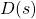, are the poles. That way its convenient to represent the Laplace transform in the complex s-plane, putting X's in the locations of poles and O's in the locations of zeroes. This pole-zero diagram can help specify the algebraic expression for the Laplace transform. Of course, the ROC must also be known.

We have three choices for the ROC, which are:

1. The ROC contains no poles,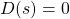2. The ROC of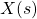consists of a strip parallel to the jω-axis in the s-plane
3. The Fourier transform of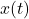converges and so ROC includes the jω-axis in the s-plane

### Example

Let's consider the Laplace transform expression:All three of the following pole-zero diagrams (and the ROC's they correspond to) are valid representations:Some additional useful rules and properties:

• For signals of finite duration, the ROC is mostly the entire s-plane
• For right-sided signals within the ROC, all values greater than σ0 are also in the ROC. Whenis rational, the ROC is basically to the right of the rightmost pole.
• Similarly, for left-sided signals within the ROC all values less than σ0 are also in the ROC, and when rational the ROC is to the left of the leftmost pole.
• For two-sides signal within the ROC, the ROC is a strip in the s-plane.

Sometimes its easier to specify the Laplace by decomposing it into a partial fraction expansion. For example, in the case offrom the previous example: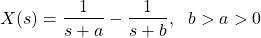Using such properties its sometimes easy to identify the ROC directly from the pole-zero pattern in the s-plane. Of course auxilary information about the signal in the time-domain has to also be known, like if the signal is right-sided, left-sided or two-sided. But, either way, the relation of the poles of the Laplace transform with certain properties of the signal in the time-domain are very important to include, while doing LTI system analysis using the Laplace transform.

## RESOURCES:

### Images

1.Block diagrams and other visualizations were made using draw.io and GeoGebra

## Final words | Next up

And this is actually it for today's post!

Next time we will get into how the Laplace Transform can be used to simplify calculations on Second-Order Systems...

See Ya!Keep on drifting!

H2
H3
H4
3 columns
2 columns
1 column
1 Comment